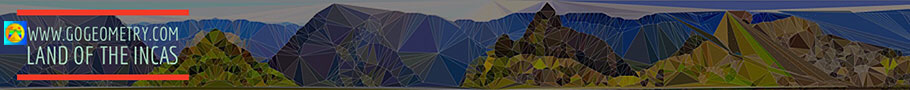# Geometry: Orthocenter of a triangle, Theorems and Problems - Index 5Geometry Problem 782 Triangle, Orthocenter, Circumcenter, Circumcircle, Diameter, Altitude, Parallelogram. Geometry Problem 781 Triangle, Orthocenter, Circumcenter, Vertex, Altitude, Midpoint, Perpendicular. Geometry Problem 780 Triangle, Sum of Inradius and Circumradius, Distances from the Orthocenter to the Vertices. Geometry Problem 779 Triangle, Altitude, Orthocenter, Midpoint, Angle, 90 Degrees. Geometry Problem 778 Triangle, Distance from the Orthocenter to a Vertex, Circle, Circumradius, Side, Square. Go to Page: Previous | 1 | 5 | 10 | Next

Home | SearchGeometry | Problems | Triangle Centers | Orthocenter | Altitude | Post a comment | Email | by Antonio Gutierrez